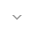# IntArrayEvaluator

`public class IntArrayEvaluator`
`extends Object` `implements TypeEvaluator<int[]>`

 java.lang.Object ↳ android.animation.IntArrayEvaluator

## Summary

### Public constructors

` IntArrayEvaluator() `

` IntArrayEvaluator(int[] reuseArray) `

### Public methods

` int[]` ` evaluate(float fraction, int[] startValue, int[] endValue) `

### Inherited methodsFrom class ` java.lang.Object `From interface ` android.animation.TypeEvaluator `

## Public constructors

### IntArrayEvaluator

`IntArrayEvaluator ()`

• `IntArrayEvaluator(int[])`

### IntArrayEvaluator

`IntArrayEvaluator (int[] reuseArray)`

Parameters
`reuseArray` `int`: The array to modify and return from `evaluate`.

## Public methods

### evaluate

```int[] evaluate (float fraction,
int[] startValue,
int[] endValue)```

Parameters
`fraction` `float`: The fraction from the starting to the ending values
`startValue` `int`: The start value.
`endValue` `int`: The end value.
Returns
`int[]` An `int[]` where each element is an interpolation between the same index in startValue and endValue.